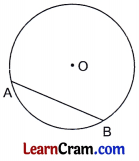# DAV Class 6 Maths Chapter 13 Worksheet 1 Solutions

The DAV Maths Book Class 6 Solutions and DAV Class 6 Maths Chapter 13 Worksheet 1 Solutions of Circles offer comprehensive answers to textbook questions.

## DAV Class 6 Maths Ch 13 WS 1 Solutions

Question 1.
Name three objects that can be used to draw a circle.
Pencil, scale and compass are the three objects that are used to draw a circle.

Question 2.
In the given figure, name the centre and all radii of the circle.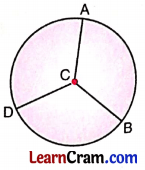(i) Centre is C
(ii) Radii are CA, CB, CD

Question 3.
Name all the radii, diameters and chords in this circle.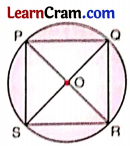(i) Radii : OP, OQ, OR and OS
(ii) Diameters : PR and QS
(iii) Chords : PQ, QR, RS, SP, PR and SQQuestion 4.
Draw the following in this circle: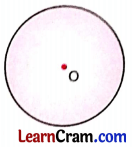(b) Diameter AB
(c) Chord AC
(a) 0 is the centre and X any point on the circle. Join OX which is the required radius of the given circle.
(b) Draw a line segment through the centre meeting the circle at A and B.
Hence, AB is the required diameter.
(c) Join any two points A and C on the circle.
Hence, AC is the required chord of the circle.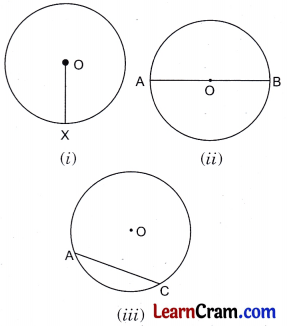Question 5.
In this circle,
(a) MN and XY are ___________
(b) XN and MY are ___________
(c) OX and OM are ___________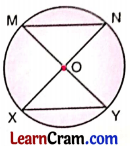(a) Chord
(b) Diameters

Question 6.
Find the diameter of circles whose radius is given below:
(a) 4 cm
∴ Diameter = 2 × Radius
= 2 × 4 = 8 cm
Hence the required diameter = 8 cm.

(b) 3.1 cm
= 2 × 3.1 = 6.2 cm
Hence, the required diameter = 6.2 cm.

(c) 4.6 cm
∴ Diameter = 2 × Radius
= 2 × 4.6 = 9.2 cm
Hence, the required diameter = 9.2 cm.

(d) 5.4 cm
∴ Diameter = 2 × Radius
= 2 × 5.4 = 10.8 cm
Hence, the required diameter = 10.8 cm

(e) 21 cm
∴ Diameter = 2 × Radius
= 2 × 21 = 42 cm
Hence, the required diameter = 42 cm.Question 7.
Diameter of some circles are given below. Find their radius.
(a) 8 cm
Here, diameter = 8 cm
∴ Radius = $$\frac{1}{2}$$ × diameter
= $$\frac{1}{2}$$ × 8 = 4 cm
Hence, the required radius = 4 cm.

(b) 7 cm
Here, diameter = 7 cm.
∴ Radius = $$\frac{1}{2}$$ × diameter
= $$\frac{1}{2}$$ × 7 = 3.5 cm
Hence, the required radius = 3.5 cm.

(c) 6.4 cm
Here, diameter = 6.4 cm.
∴ Radius = $$\frac{1}{2}$$ × diameter
= $$\frac{1}{2}$$ × 6.4 = 3.2 cm
Hence, the required radius = 3.2 cm.

(d) 11.4 cm
Here, diameter = 11.4 cm.
∴ Radius = $$\frac{1}{2}$$ × diameter
= $$\frac{1}{2}$$ × 11.4 = 5.7 cm
Hence, the required radius = 5,7 cm.

(e) 26 cm
Here, diameter = 26 cm.
∴ Radius = $$\frac{1}{2}$$ × diameter
= $$\frac{1}{2}$$ × 26 = 13 cm
Hence, the required radius = 13 cm.

Question 8.
Observe the given circle and answer the following questions.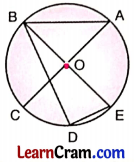(a) Name the centre.
O is the centre of the given circle.

(b) How many radii are there?

(c) Name any one diameter.
AC is the diameter of the circle.

(d) Name all the chords.
AC, BE, BD, AB and DE are the chords of the circle.

(e) Name a semi-circle.
ABC is a semicircle with centre O and diameter AC.Question 9.
Write ‘True’ or ‘False’ for the following statements:
(a) Diameter of a circle is twice its radius.
True

(b) The line segment joining any two points on a circle is called its diameter.
False

(c) All the radii of a circle are of equal length.
True

(d) We can draw only two diameters of a circle.
False

(e) Each circle can have only two semi¬circles.
True

### DAV Class 6 Maths Chapter 13 Worksheet 1 Notes

Elements of Circle
1.Centre:
It is a central point of a circle whose distance from every point on the circle remains same.

The shortest distance of the centre to any point on the circle is called radius of the circle.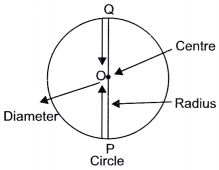3.Diameter:
It is twice the length of the radius. Diameter = 2 x Radius

4.Semicircle:
Diameter of the circle divides it into two equal halves which are known as semicircles.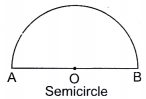5.Chord:
It is a line segment obtained by joining any two points on the circle.
Here, AB is the chord of the circle with centre O.
Diameter is the longest chord of the circle.

6.Circumference:
Perimeter of a circle is called its circumference.